%config InlineBackend.figure_format = 'retina'
import pymc3 as pm

Markov Models From The Bottom Up, with Python

Markov models are a useful class of models for sequential-type of data. Before recurrent neural networks (which can be thought of as an upgraded Markov model) came along, Markov Models and their variants were the in thing for processing time series and biological data.

Just recently, I was involved in a project with a colleague, Zach Barry, where we thought the use of autoregressive hidden Markov models (AR-HMMs) might be a useful thing. Apart from our hack session one afternoon, it set off a series of self-study that culminated in this essay. By writing this down for my own memory, my hope is that it gives you a resource to refer back to as well.

You'll notice that I don't talk about inference (i.e. inferring parameters from data) until the end: this is intentional. As I've learned over the years doing statistical modelling and machine learning, nothing makes sense without first becoming deeply familiar with the "generative" story of each model, i.e. the algorithmic steps that let us generate data. It's a very Bayesian-influenced way of thinking that I hope you will become familiar with too.

Markov Models: What they are, with mostly plain English and some math

The simplest Markov models assume that we have a system that contains a finite set of states, and that the system transitions between these states with some probability at each time step $t$, thus generating a sequence of states over time. Let's call these states $S$, where

S = \{s_1, s_2, ..., s_n\}

S = \{s_1, s_2, s_3\}

A Markov model generates a sequence of states, with one possible realization being:

\{s_1, s_1, s_1, s_3, s_3, s_3, s_2, s_2, s_3, s_3, s_3, s_3, s_1, ...\}

And generically, we represent it as a sequence of states $x_t, x_{t+1}... x_{t+n}$. (We have chosen a different symbol to not confuse the "generic" state with the specific realization. Graphically, a plain and simple Markov model looks like the following: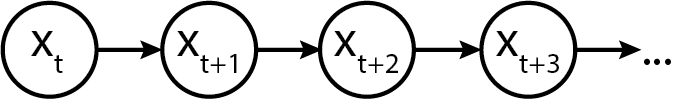Initializing a Markov chain

Every Markov chain needs to be initialized. To do so, we need an initial state probability vector, which tells us what the distribution of initial states will be. Let's call the vector $p_S$, where the subscript $S$ indicates that it is for the "states".

p_{init} = \begin{pmatrix} p_1 & p_2 & p_3 \end{pmatrix}

Semantically, they allocate the probabilities of starting the sequence at a given state. For example, we might assume a discrete uniform distribution, which in Python would look like:

import numpy as np
p_init = np.array([1/3., 1/3., 1/3.])

Alternatively, we might assume a fixed starting point, which can be expressed as the $p_S$ array:

p_init = np.array([0, 1, 0])

Alternatively, we might assign non-zero probabilities to each in a non-uniform fashion:

# State 0: 0.1 probability
# State 1: 0.8 probability
# State 2: 0.1 probability
p_init = np.array([0.1, 0.8, 0.1])

Finally, we might assume that the system was long-running before we started observing the sequence of states, and as such the initial state was drawn as one realization of some equilibrated distribution of states. Keep this idea in your head, as we'll need it later.

For now, just to keep things concrete, let's specify an initial distribution as a non-uniform probability vector.

import numpy as np
p_init = np.array([0.1, 0.8, 0.1])

Modelling transitions between states

To know how a system transitions between states, we now need a transition matrix. The transition matrix describes the probability of transitioning from one state to another. (The probability of staying in the same state is semantically equivalent to transitioning to the same state.)

By convention, transition matrix rows correspond to the state at time $t$, while columns correspond to state at time $t+1$. Hence, row probabilities sum to one, because the probability of transitioning to the next state depends on only the current state, and all possible states are known and enumerated.

Let's call the transition matrix $P_{transition}$. The symbol etymology, which usually gets swept under the rug in mathematically-oriented papers, are as follows:

• $transition$ doesn't refer to time but simply indicates that it is for transitioning states,
• $P$ is used because it is a probability matrix.
P_{transition} = \begin{pmatrix} p_{11} & p_{12} & p_{13}\\ p_{21} & p_{22} & p_{23}\\ p_{31} & p_{32} & p_{33}\\ \end{pmatrix}

Using the transition matrix, we can express that the system likes to stay in the state that it enters into, by assigning larger probability mass to the diagonals. Alternatively, we can express that the system likes to transition out of states that it enters into, by assigning larger probability mass to the off-diagonal.

Alrighty, enough with that now, let's initialize a transition matrix below.

p_transition = np.array(
[[0.90, 0.05, 0.05],
[0.01, 0.90, 0.09],
[0.07, 0.03, 0.9]]
)
p_transition
array([[0.9 , 0.05, 0.05],
[0.01, 0.9 , 0.09],
[0.07, 0.03, 0.9 ]])

And just to confirm with you that each row sums to one:

assert p_transition[0, :].sum() == 1
assert p_transition[1, :].sum() == 1
assert p_transition[2, :].sum() == 1

Equilibrium or Stationary Distribution

Now, do you remember how above we talked about the Markov chain being in some "equilibrated" state? Well, the stationary or equilibrium distribution of a Markov chain is the distribution of observed states at infinite time.

An interesting property is that regardless of what the initial state is, the equilibrium distribution will always be the same, as the equilibrium distribution only depends on the transition matrix.

Here's how to think about the equilibrium: if you were to imagine instantiating a thousand Markov chains using the initial distribution

p_{init} = \begin{pmatrix} 0.1 & 0.8 & 0.1 \end{pmatrix}
• 10% would start out in state 1
• 80% would start out in state 2
• 10% would start out in state 3

However, if you ran each of the systems to a large number of time steps (say, 1 million time steps, to exaggerate the point) then how the states were distributed initially wouldn't matter, as how they transition from time step to time step begins to dominate.

We could simulate this explicitly in Python, but as it turns out, there is a mathematical shortcut that invovles simple dot products. Let's check it out.

Assume we have an initial state and a transition matrix. We're going to reuse p_init from above, but use a different p_transition to make the equilibrium distribution values distinct. This will make it easier for us to plot later.

p_transition_example = np.array(
[[0.6,  0.2, 0.2],
[0.05, 0.9, 0.05],
[0.1,  0.2, 0.7]]
)

To simulate the distribution of states in the next time step, we take the initial distribution p_init and matrix multiply it against the transition matrix.

p_next = p_init @ p_transition_example
p_next
array([0.11, 0.76, 0.13])

We can do it again to simulate the distribution of states in the next time step after:

p_next = p_next @ p_transition_example
p_next
array([0.117, 0.732, 0.151])

Let's now write a for-loop to automate the process.

p_state_t = [p_init]

for i in range(200):  # 200 time steps sorta, kinda, approximates infinite time :)
p_state_t.append(p_state_t[-1] @ p_transition_example)

To make it easier for you to see what we've generated, let's make the p_state_t list into a pandas DataFrame.

import pandas as pd

state_distributions = pd.DataFrame(p_state_t)
state_distributions
0 1 2
0 0.100000 0.800000 0.10000
1 0.110000 0.760000 0.13000
2 0.117000 0.732000 0.15100
3 0.121900 0.712400 0.16570
4 0.125330 0.698680 0.17599
... ... ... ...
196 0.133333 0.666667 0.20000
197 0.133333 0.666667 0.20000
198 0.133333 0.666667 0.20000
199 0.133333 0.666667 0.20000
200 0.133333 0.666667 0.20000

201 rows × 3 columns

Now, let's plot what the distributions look like.

import matplotlib.pyplot as plt
state_distributions.plot();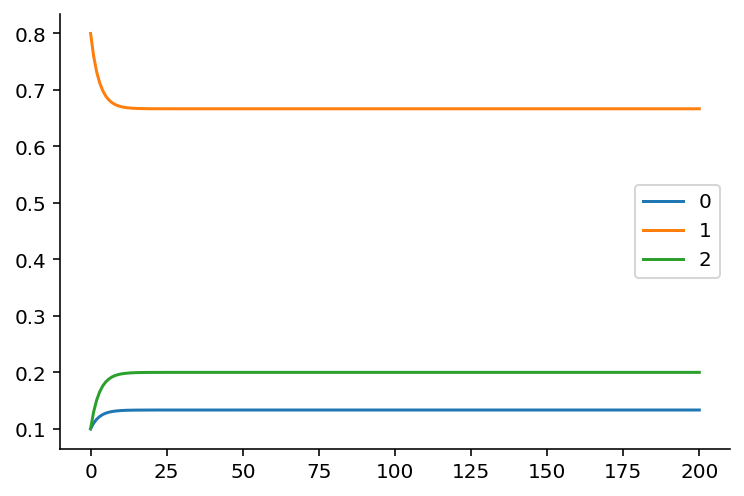If you're viewing this notebook on Binder or locally, go ahead and modify the initial state to convince yourself that it doesn't matter what the initial state will be: the equilibrium state distribution, which is the fraction of time the Markov chain is in that state over infinite time, will always be the same as long as the transition matrix stays the same.

print(p_state_t[-1])
[0.13333333 0.66666667 0.2       ]

As it turns out, there's also a way to solve for the equilibrium distribution analytically from the transition matrix. This involves solving a linear algebra problem, which we can do using Python. (Credit goes to this blog post from which I modified the code to fit the variable naming here.)

def equilibrium_distribution(p_transition):
n_states = p_transition.shape
A = np.append(
arr=p_transition.T - np.eye(n_states),
values=np.ones(n_states).reshape(1, -1),
axis=0
)
b = np.transpose(np.array( * n_states + ))
p_eq = np.linalg.solve(
a=np.transpose(A).dot(A),
b=np.transpose(A).dot(b)
)
return p_eq

# alternative
def equilibrium_distribution(p_transition):
"""This implementation comes from Colin Carroll, who kindly reviewed the notebook"""
n_states = p_transition.shape
A = np.append(
arr=p_transition.T - np.eye(n_states),
values=np.ones(n_states).reshape(1, -1),
axis=0
)
# Moore-Penrose pseudoinverse = (A^TA)^{-1}A^T
pinv = np.linalg.pinv(A)
# Return last row
return pinv.T[-1]

print(equilibrium_distribution(p_transition_example))
[0.13333333 0.66666667 0.2       ]

Generating a Markov Sequence

Generating a Markov sequence means we "forward" simulate the chain by:

(1) Optionally drawing an initial state from $p_S$ (let's call that $s_{t}$). This is done by drawing from a multinomial distribution:

s_t \sim Multinomial(1, p_S)

If we assume (and keep in mind that we don't have to) that the system was equilibrated before we started observing its state sequence, then the initial state distribution is equivalent to the equilibrium distribution. All this means that we don't necessarily have to specify the initial distribution explicitly.

(2) Drawing the next state by indexing into the transition matrix $p_T$, and drawing a new state based on the Multinomial distribution:

s_{t+1} \sim Multinomial(1, p_{T_i})

where $i$ is the index of the state.

I previously wrote about what probability distributions are, leveraging the SciPy probability distributions library. We're going to use that extensively here, as opposed to NumPy's random module, so that we can practice getting familiar with probability distributions as objects. In Python code:

from scipy.stats import multinomial
from typing import List

def markov_sequence(p_init: np.array, p_transition: np.array, sequence_length: int) -> List[int]:
"""
Generate a Markov sequence based on p_init and p_transition.
"""
if p_init is None:
p_init = equilibrium_distribution(p_transition)
initial_state = list(multinomial.rvs(1, p_init)).index(1)

states = [initial_state]
for _ in range(sequence_length - 1):
p_tr = p_transition[states[-1]]
new_state = list(multinomial.rvs(1, p_tr)).index(1)
states.append(new_state)
return states

With this function in hand, let's generate a sequence of length 1000.

import seaborn as sns

states = markov_sequence(p_init, p_transition, sequence_length=1000)
fig, ax = plt.subplots(figsize=(12, 4))
plt.plot(states)
plt.xlabel("time step")
plt.ylabel("state")
plt.yticks([0, 1, 2])
sns.despine()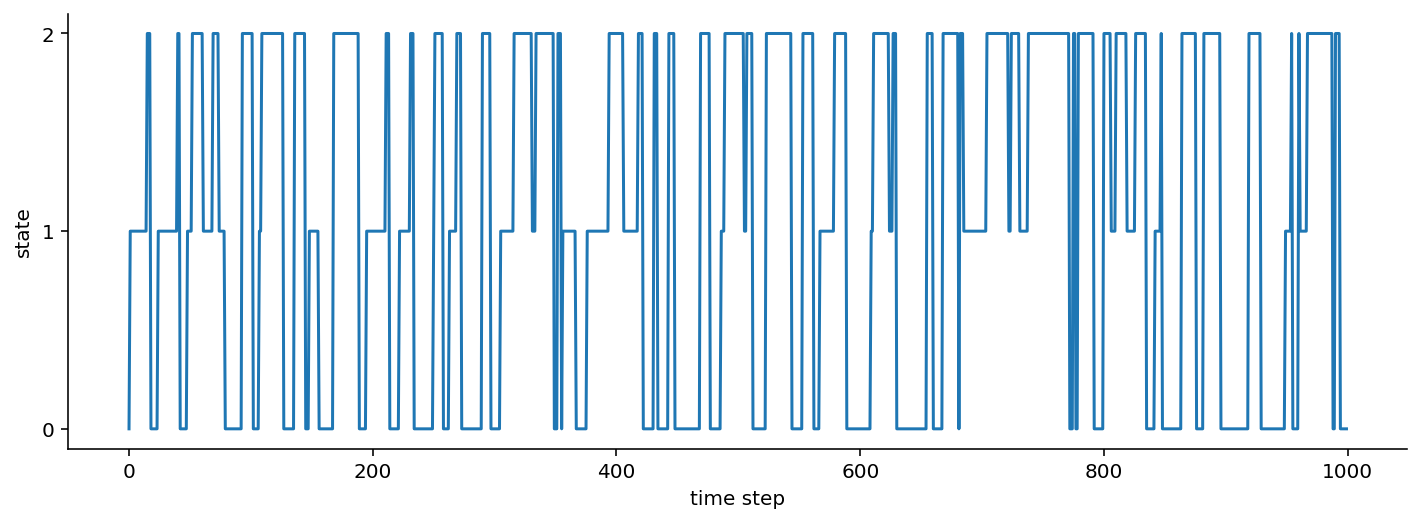As is pretty evident from the transition probabilities, once this Markov chain enters a state, it tends to maintain its current state rather than transitioning between states.

If you've opened up this notebook in Binder or locally, feel free to modify the transition probabilities and initial state probabilities above to see how the Markov sequence changes.

If a "Markov sequence" feels abstract at this point, one example to help you anchor your understanding would be human motion. The three states can be "stationary", "walking", and "running". We transition between the three states with some probability throughout the day, moving from "stationary" (sitting at my desk) to "walking" (to get water) to "stationary" (because I'm pouring water), to "walking" (out the door) to finally "running" (for exercise).

Emissions: When Markov chains not only produce "states", but also observable data

So as you've seen above, a Markov chain can produce "states". If we are given direct access to the "states", then a problem that we may have is inferring the transition probabilities given the states.

A more common scenario, however, is that the states are latent, i.e. we cannot directly observe them. Instead, the latent states generate data that are given by some distribution conditioned on the state. We call these Hidden Markov Models.

That all sounds abstract, so let's try to make it more concrete.

Gaussian Emissions: When Markov chains emit Gaussian-distributed data.

With a three state model, we might say that the emissions are Gaussian distributed, but the location ($\mu$) and scale ($\sigma$) vary based on which state we are in. In the simplest case:

1. State 1 gives us data $y_1 \sim N(\mu=1, \sigma=0.2)$
2. State 2 gives us data $y_2 \sim N(\mu=0, \sigma=0.5)$
3. State 3 gives us data $y_3 \sim N(\mu=-1, \sigma=0.1)$

In terms of a graphical model, it would look something like this: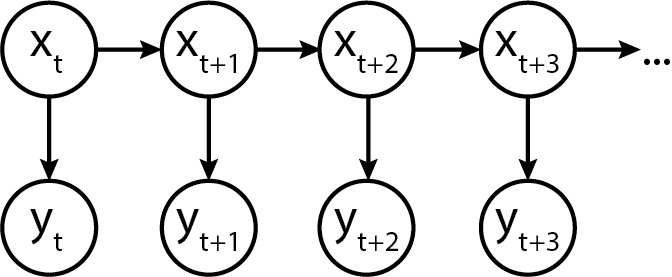Turns out, we can model this in Python code too!

from scipy.stats import norm

def gaussian_emissions(states: List[int], mus: List[float], sigmas: List[float]) -> List[float]:
emissions = []
for state in states:
loc = mus[state]
scale = sigmas[state]
e = norm.rvs(loc=loc, scale=scale)
emissions.append(e)
return emissions

Let's see what the emissions look like.

gaussian_ems = gaussian_emissions(states, mus=[1, 0, -1], sigmas=[0.2, 0.5, 0.1])

def plot_emissions(states, emissions):
fig, axes = plt.subplots(figsize=(16, 8), nrows=2, ncols=1, sharex=True)

axes.plot(states)
axes.set_title("States")
axes.plot(emissions)
axes.set_title("Emissions")
sns.despine();

plot_emissions(states, gaussian_ems)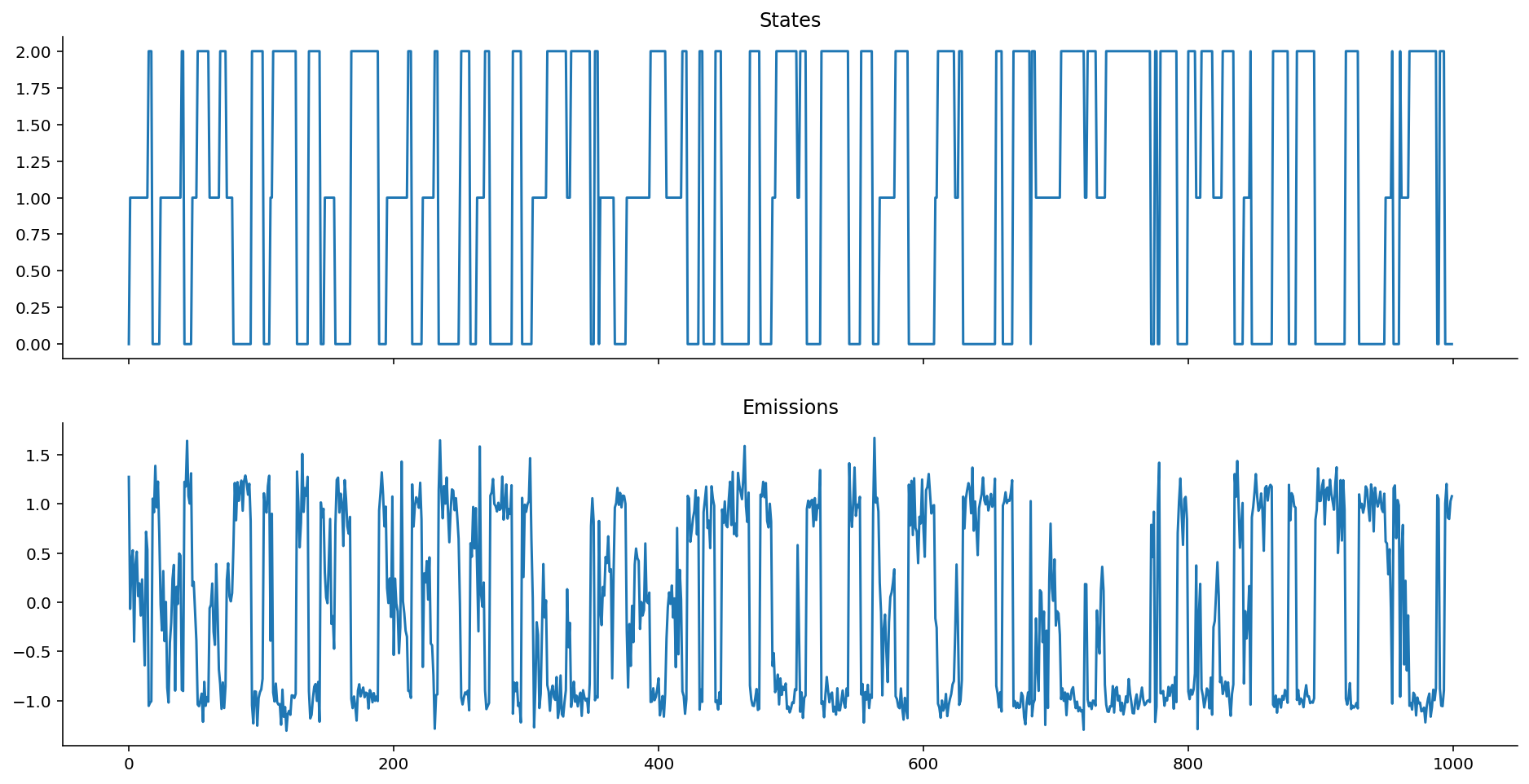Emission Distributions can be any valid distribution!

Nobody said we have to use Gaussian distributions for emissions; we can, in fact, have a ton of fun and start simulating data using other distributions!

Let's try Poisson emissions. Here, then, the poisson rate $\lambda$ is given one per state. In our example below:

1. State 1 gives us data $y_1 \sim Pois(\lambda=1)$
2. State 2 gives us data $y_2 \sim Pois(\lambda=10)$
3. State 3 gives us data $y_3 \sim Pois(\lambda=50)$
from scipy.stats import poisson
def poisson_emissions(states: List[int], lam: List[float]) -> List[int]:
emissions = []
for state in states:
rate = lam[state]
e = poisson.rvs(rate)
emissions.append(e)
return emissions

Once again, let's observe the emissions:

poisson_ems = poisson_emissions(states, lam=[1, 10, 50])
plot_emissions(states, poisson_ems)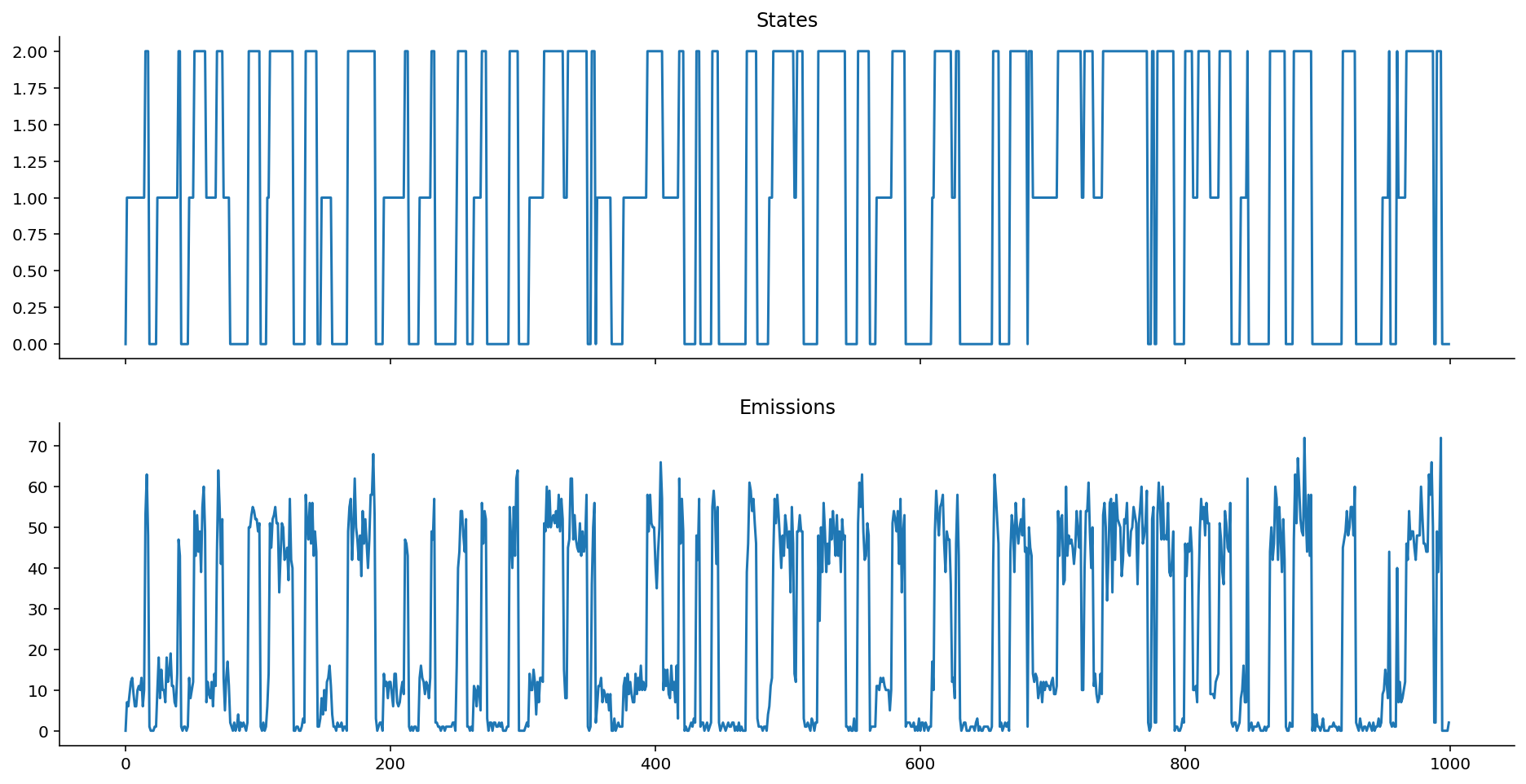Hope the point is made: Take your favourite distribution and use it as the emission distribution, as long as it can serve as a useful model for the data that you observe!

Autoregressive Emissions

Autoregressive emissions make things even more interesting and flexible! They show up, for example, when we're trying to model "motion states" of people or animals: that's because people and animals don't abruptly change from one state to another, but gradually transition in.

The "autoregressive" component thus helps us model that the emission value does not only depend on the current state, but also on previous state(s), which is what motion data, for example, might look like.

How, though, can we enforce this dependency structure? Well, as implied by the term "structure", it means we have some set of equations that relate the parameters of the emission distribution to the value of the previous emission.

In terms of a generic graphical model, it is represented as follows: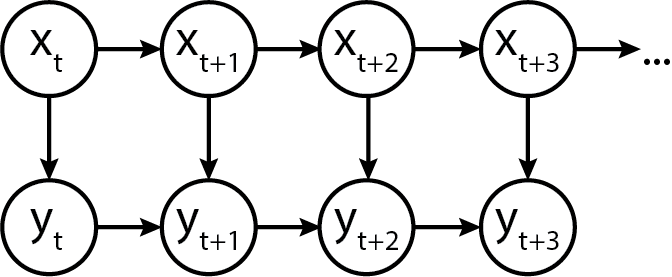Heteroskedastic Autoregressive Emissions

Here's a "simple complex" example, where the location $\mu_t$ of the emission distribution at time $t$ depends on $y_{t-1}$, and the scale $\sigma$ depends only on the current state $s_t$.

A place where this model might be useful is when we believe that noise is the only thing that depends on state, while the location follows a random walk. (Stock markets might be an applicable place for this.)

In probabilistic notation:

y_t \sim N(\mu=k y_{t-1}, \sigma=\sigma_{s_t})

Here, $k$ is a multiplicative autoregressive coefficient that scales how the previous emission affects the location $\mu$ of the current emission. We might also assume that the initial location $\mu=0$. Because the scale $\sigma$ varies with state, the emissions are called heteroskedastic, which means "of non-constant variance". In the example below:

1. State 1 gives us $\sigma=0.5$ (kind of small variance).
2. State 2 gives us $\sigma=0.1$ (smaller variance).
3. State 3 gives us $\sigma=0.01$ (very small varaince).

In Python code, we would model it this way:

def ar_gaussian_heteroskedastic_emissions(states: List[int], k: float, sigmas: List[float]) -> List[float]:
emissions = []
prev_loc = 0
for state in states:
e = norm.rvs(loc=k * prev_loc, scale=sigmas[state])
emissions.append(e)
prev_loc = e
return emissions
ar_het_ems = ar_gaussian_heteroskedastic_emissions(states, k=1, sigmas=[0.5, 0.1, 0.01])
plot_emissions(states, ar_het_ems)